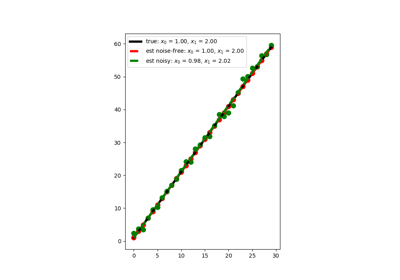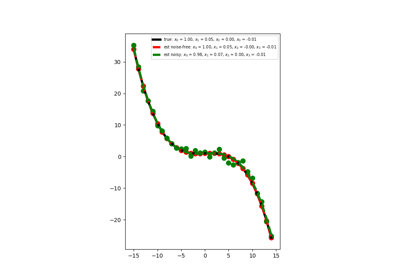# pylops.optimization.sparsity.IRLS¶

pylops.optimization.sparsity.IRLS(Op, data, nouter, threshR=False, epsR=1e-10, epsI=1e-10, x0=None, tolIRLS=1e-10, returnhistory=False, **kwargs_cg)[source]

Iteratively reweighted least squares.

Solve an optimization problem with $$L1$$ cost function given the operator Op and data y. The cost function is minimized by iteratively solving a weighted least squares problem with the weight at iteration $$i$$ being based on the data residual at iteration $$i+1$$.

The IRLS solver is robust to outliers since the L1 norm given less weight to large residuals than L2 norm does.

Parameters: Op : pylops.LinearOperator Operator to invert data : numpy.ndarray Data nouter : int Number of outer iterations threshR : bool, optional Apply thresholding in creation of weight (True) or damping (False) epsR : float, optional Damping to be applied to residuals for weighting term espI : float, optional Tikhonov damping x0 : numpy.ndarray, optional Initial guess tolIRLS : float, optional Tolerance. Stop outer iterations if difference between inverted model at subsequent iterations is smaller than tolIRLS returnhistory : bool, optional Return history of inverted model for each outer iteration of IRLS **kwargs_cg Arbitrary keyword arguments for scipy.sparse.linalg.cg solver xinv : numpy.ndarray Inverted model nouter : int Number of effective outer iterations xinv_hist : numpy.ndarray, optional History of inverted model rw_hist : numpy.ndarray, optional History of weights

Notes

Solves the following optimization problem for the operator $$\mathbf{Op}$$ and the data $$\mathbf{d}$$:

$J = ||\mathbf{d} - \mathbf{Op} \mathbf{x}||_1$

by a set of outer iterations which require to repeateadly solve a weighted least squares problem of the form:

$\mathbf{x}^{(i+1)} = \operatorname*{arg\,min}_\mathbf{x} ||\mathbf{d} - \mathbf{Op} \mathbf{x}||_{2, \mathbf{R}^{(i)}} + \epsilon_I^2 ||\mathbf{x}||$

where $$\mathbf{R}^{(i)}$$ is a diagonal weight matrix whose diagonal elements at iteration $$i$$ are equal to the absolute inverses of the residual vector $$\mathbf{r}^{(i)} = \mathbf{y} - \mathbf{Op} \mathbf{x}^{(i)}$$ at iteration $$i$$. More specifically the j-th element of the diagonal of $$\mathbf{R}^{(i)}$$ is

$R^{(i)}_{j,j} = \frac{1}{|r^{(i)}_j|+\epsilon_R}$

or

$R^{(i)}_{j,j} = \frac{1}{max(|r^{(i)}_j|, \epsilon_R)}$

depending on the choice threshR. In either case, $$\epsilon_R$$ is the user-defined stabilization/thresholding factor .

## Examples using pylops.optimization.sparsity.IRLS¶Linear RegressionPolynomial Regression